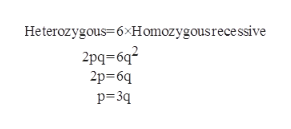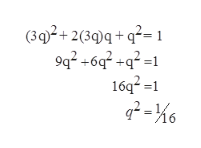You are studying a single-gene locus with two alleles in a population that is in Hardy–Weinberg equilibrium. Examination of a large sample of individuals from the population reveals there are six times as many heterozygotes as there are homozygote recessive individuals in this population. What is the frequency of the recessive allele?I don't know how to start his calculation with just a ratio of 6:1 I found an answer online that says the frequency of q=0.25 but the calculations (below) don't make sense to me. Please help me. What is the answer and how do I get to it?Hardy-Weinberg equation p2+2pq+q2=12pq/q2= 6/12p= 6q2(1-p)= 6q2-2q=6qq=0.25

Question

You are studying a single-gene locus with two alleles in a population that is in Hardy–Weinberg equilibrium. Examination of a large sample of individuals from the population reveals there are six times as many heterozygotes as there are homozygote recessive individuals in this population. What is the frequency of the recessive allele?

I don't know how to start his calculation with just a ratio of 6:1 I found an answer online that says the frequency of q=0.25 but the calculations (below) don't make sense to me. Please help me. What is the answer and how do I get to it?

Hardy-Weinberg equation p2+2pq+q2=1

2pq/q2= 6/1

2p= 6q

2(1-p)= 6q

2-2q=6q

q=0.25

Step 1

In the Hardy-Weinberg equilibrium, the allele frequency and the genotype frequency of the individuals remain the same for the various generations. According to the Hardy-Weinberg equation:

p2 + 2pq + q2 = 1.

Where,

p2 = Frequency of the homozygous genotype (dominant)

q2 = Frequency of the homozygous genotype (recessive)

2pq = Frequency of the heterozygous genotype

Step 2

Here, it is given that heterozygous individuals are 6 times more than homozygous recessive. So the ratio for heterozygous: homozygous recessive is 6:1.help_outlineImage TranscriptioncloseHeterozygous=6xHomozygousrece ssive 2pq-6q2 2p 6q p 3q fullscreen
Step 3

According to the Hardy...help_outlineImage Transcriptionclose(302+230q+q2= 9q2 +6 +q2= 16q21 2-6 =1 fullscreen

Want to see the full answer?

See Solution

Want to see this answer and more?

Our solutions are written by experts, many with advanced degrees, and available 24/7

See Solution
Tagged in

Genetics